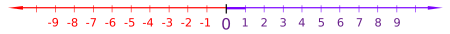abstractmath.org 2.0
help with abstract math

Produced by Charles Wells   Revised 2015-12-24

Introduction to this website  website TOC  website index  blog



# INTEGERS

An integer is any whole number. An integer can be zero, greater than zero or less than zero.  The integers greater than zero are the natural numbers. I will not give a formal definition of integer here but the Wikipedia article gives one. This article describes their most elementary properties and points out some sources of confusion.

## Properties of integers

### Closure

If $m$ and $n$ are integers, then so are $m+n$, $m–n$ and $mn.$

This is described by saying that the integers are closed under addition, subtraction and multiplication. They are not closed under division. For example, $3$ and $5$ are integers but $3/5$ is not an integer.

Compare:

• The natural numbers are closed under addition and multiplication, but not under subtraction.
• The rational numbers are closed under addition, subtraction and multiplication, and under division by any nonzero number.

### Positive and negative

For any integer $n$:

• $n$ is positive if $n\gt0.$
• $n$ is negative if $n\lt0.$
• $n$ is nonnegative if $n\geq0.$

Some things to pay attention to:

• "$n$ is not positive" does not mean the same thing as "$n$ is negative".
• $0$ is neither positive nor negative.

In some countries, schools may use “positive” to mean “nonnegative”, so that zero is positive. Occasionally, students have told me that their high school teachers said $0$ is both positive and negative.

## Images and metaphors for integers

I will give two ways it is useful to think about integers.  You will no doubt be familiar with them.  They are included here partly to illustrate the ways in which we think about mathematical objects using images and metaphors.  (In other words, in this section, I am not teaching you only about the integers but also about images and metaphors!)

### Ordered list infinite in both directions

• The positive integers increase in size as you move to the right and the negative integers increase in size as you move to the left:
•• So in contrast to the natural numbers, the integers form a list that is infinite in both directions.
• Adding a positive number $n$ to another one takes you to the number that is $n$ spaces to the right.  Adding a negative number $-n$ takes you to the number that is $n$ spaces to the left.
A negative integer such as $-5$ does not have an immediately obvious interpretation as the number of elements of a set.  One interpretation that does make sense of negative integers involves credit and debit.  If you have $n$ dollars (for $n$ positive) that is what you have. Having $-n$ dollars represents the fact that you owe $n$ dollars.